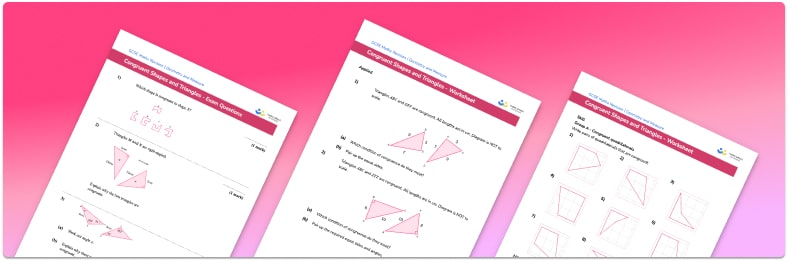# Congruent Shapes Worksheets• Section 1 of the congruent shapes worksheet contains 36 skills-based congruent shapes questions, in 3 groups to support differentiation
• Section 2 contains 4 applied congruent shapes questions with a mix of word problems and deeper problem solving questions
• Section 3 contains 4 foundation and higher level GCSE exam style congruent shapes questions
• Answer keys and a mark scheme for all congruent shapes questions are provided
• Questions follow variation theory with plenty of opportunities for students to work independently at their own level
• All questions created by fully qualified expert secondary maths teachers
• Suitable for GCSE maths revision for AQA, OCR and Edexcel exam boards

• This field is for validation purposes and should be left unchanged.

You can unsubscribe at any time (each email we send will contain an easy way to unsubscribe). To find out more about how we use your data, see our privacy policy.

### Congruent shapes at a glance

Congruent shapes are 2d shapes which are exactly the same shape and size. In order to identify pairs of congruent shapes each of the corresponding sides of the shapes must be the same and each of the corresponding angles of the shapes must be the same (although they may be flipped or rotated).

Whilst congruent figures can be any 2d shape including triangles, quadrilaterals and polygons, there are some special rules which help us identify congruent triangles.

In pairs of triangles, congruence can be proved if any of the following criteria can be met: SSS (all three sides of the two triangles are equal), ASA (two angles and the side between them are equal), SAS (two sides and the angle between them are equal), RHS (The triangles both have right angles, the hypotenuses are equal and one other side is equal).

Congruence differs from mathematical similarity in that we define similar shapes as shapes which are the same shape but not necessarily the same size whereas congruent shapes are exactly the same shape and size.

Looking forward, students can then progress to additional geometry worksheets, for example a 3D shapes worksheet or an.For more teaching and learning support on Geometry our GCSE maths lessons provide step by step support for all GCSE maths concepts.

## Do you have KS4 students who need more focused attention to succeed at GCSE?There will be students in your class who require individual attention to help them succeed in their maths GCSEs. In a class of 30, it’s not always easy to provide.

Help your students feel confident with exam-style questions and the strategies they’ll need to answer them correctly with our dedicated GCSE maths revision programme.

Lessons are selected to provide support where each student needs it most, and specially-trained GCSE maths tutors adapt the pitch and pace of each lesson. This ensures a personalised revision programme that raises grades and boosts confidence.

Find out more